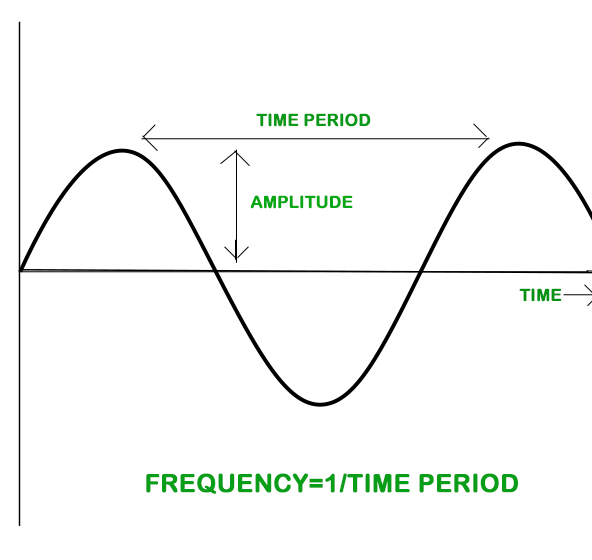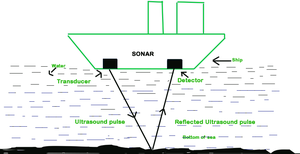Related Articles

# Amplitude, Time Period and Frequency of a Vibration

• Last Updated : 26 May, 2021

Sound is a form of energy generated by vibrating bodies. Its spread necessitates the use of a medium. As a result, sound cannot travel in a vacuum because there is no material to transfer sound waves. Sound vibration is the back and forth motion of an entity that causes the sound to be made. That is often referred to as oscillatory motion

### What are the Oscillations?

The oscillation is the usual rhythmic back and forth movement. Sound travels from one location to another in the form of waves, which are caused by the movement of objects in the medium. A wave is a phenomenon or disruption in which energy is transmitted from one point to another without physical interaction. As a result, the sound is regarded as a wave.

Attention reader! All those who say programming isn't for kids, just haven't met the right mentors yet. Join the  Demo Class for First Step to Coding Course, specifically designed for students of class 8 to 12.

The students will get to learn more about the world of programming in these free classes which will definitely help them in making a wise career choice in the future.

Each vibration or oscillation has three main characteristics: Amplitude, time period, and frequency are discussed deeply in the below section:### Amplitude

The amplitude of a sound wave is a measurement of the wave’s height. The amplitude of a sound wave is known as its loudness or the maximum displacement of vibrating particles in a medium from their mean location when the sound is made. It is the difference between the wave’s crest or trough and its mean location.

The amplitude of the wave is the frequency of the maximum disruption of particles in the medium on either side of the mean value.

The letter A is commonly used to represent it.

The SI unit for it is meter (m).

### Time Period

A time period may be described as the amount of time it takes to complete a task. When an occurrence happens on a regular basis, it is said to be intermittent. The cycle is the amount of time it takes for the seasonal phenomenon to replicate itself. The time it takes the particle to complete one vibration loop is the particle’s time span.

The time it takes to complete one vibration in the depth of the medium is referred to as the sound wave’s time cycle.

The relation between time period (T) and frequency (f) is,

T=1 / f

It is denoted by the letter T

Its SI unit is the second (s).

### Frequency

The frequency of oscillation is defined as the number of oscillations per second. Its unit is hertz, which is denoted by the symbol Hz. In general, the frequency of a wave refers to how often the particles in a medium vibrate as a wave passes through the medium.

The frequency of a sound wave is defined as the number of vibrations per unit of time.

Frequency (f) = 1 / Time Period (T)

It is represented by the letters f or v.

The SI unit of frequency is Hertz (Hz).

### Sample Problems

Problem 1: Why does an empty vessel produce a louder sound than a filled one?

Solution:

An empty vessel produces a louder sound than a filled one because the air molecules in the filled vessel have greater amplitude and greater intensity than the liquid molecules in the filled vessel.

Problem 2: What type of wave is sound?

Solution:

Sound waves fall into three categories:

1.Longitudinal Sound Waves: A longitudinal wave is a wave in which the motion of the medium’s particles is parallel to the direction of the energy transport.

2. Mechanical Sound Waves: A mechanical wave is a wave that depends on the oscillation of matter, meaning that it transfers energy through a medium to propagate

3.Pressure Sound Waves: A pressure wave, or compression wave, has a regular pattern of high- and low-pressure regions. Because sound waves consist of compressions and rarefactions, their regions fluctuate between low and high-pressure patterns.

Problem 3: A person is listening to a tone of 600 Hz sitting at a distance of 550 m from the source of the sound. What is the time interval between successive compressions from the source?

Solution:

The time interval between two successive compressions is equal to the time period of the wave. This time period is reciprocal of the frequency of the wave and is given by the relation

T =1 / f

= 1 / 600 Hz

= 1.66 ms

Problem 4: Explain the working and application of a SONAR.

Solution:An Ultrasonic sound wave is produced by the transducer of the SONAR, which propagates through any medium (seawater). The wave when hits a surface, is reflected and detected by the sonar detector. The distance of the surface can be calculated as:

2d = v × t

where d is distance, v is velocity of sound, t is time.

This method of measuring distance is also known as ‘echo-ranging’. The result is 2d because the wave covers d distance when outgoing and d distance again when coming back after reflection.

Problem 5: Describe how compressions and rarefactions are produced in the air near a source of the sound.

Solution:

Compression is a region of high pressure where particles are close while rarefaction is a region where particle is far from each other. When a wave moves forward it is compressed because of pressure of the medium. Similarly, rarefactions are seen when the wave moves backward. As the wave in air hits many particles and moves back and forth, compression and rarefactions are produced.

My Personal Notes arrow_drop_up